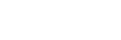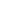Explore all Topic Modeling open source software, libraries, packages, source code, cloud functions and APIs.

## Popular New Releases in Topic Modeling

 gensim BERTopic v0.9.4 Top2Vec Phrases and new embedding options tomotopy 0.12.0 Palmetto Version 0.1.3
 gensim BERTopicv0.9.4 Top2VecPhrases and new embedding options tomotopy0.12.0 PalmettoVersion 0.1.3

1

8 Libraries

811

2

8 Libraries

762

3

6 Libraries

92

4

3 Libraries

45

5

3 Libraries

458

6

3 Libraries

72

7

3 Libraries

246

8

3 Libraries

111

9

2 Libraries

21

10

2 Libraries

9

1

8 Libraries

811

2

8 Libraries

762

3

6 Libraries

92

4

3 Libraries

45

5

3 Libraries

458

6

3 Libraries

72

7

3 Libraries

246

8

3 Libraries

111

9

2 Libraries

21

10

2 Libraries

9

## Trending Discussions on Topic Modeling

QUESTION

Display document to topic mapping after LSI using Gensim

Asked 2022-Feb-22 at 19:27

I am new to using LSI with Python and Gensim + Scikit-learn tools. I was able to achieve topic modeling on a corpus using LSI from both the Scikit-learn and Gensim libraries, however, when using the Gensim approach I was not able to display a list of documents to topic mapping.

Here is my work using Scikit-learn LSI where I successfully displayed document to topic mapping:

``````1tfidf_transformer = TfidfTransformer()
2transformed_vector = tfidf_transformer.fit_transform(transformed_vector)
3NUM_TOPICS = 14
4lsi_model = TruncatedSVD(n_components=NUM_TOPICS)
5lsi= nmf_model.fit_transform(transformed_vector)
6
7topic_to_doc_mapping = {}
8topic_list = []
9topic_names = []
10
11for i in range(len(dbpedia_df.index)):
12    most_likely_topic =  nmf[i].argmax()
13
14    if most_likely_topic not in topic_to_doc_mapping:
15        topic_to_doc_mapping[most_likely_topic] = []
16
17    topic_to_doc_mapping[most_likely_topic].append(i)
18
19    topic_list.append(most_likely_topic)
20    topic_names.append(topic_id_topic_mapping[most_likely_topic])
21
22dbpedia_df['Most_Likely_Topic'] = topic_list
23dbpedia_df['Most_Likely_Topic_Names'] = topic_names
24
25print(topic_to_doc_mapping[:100])
26
27topic_of_interest = 1
28doc_ids = topic_to_doc_mapping[topic_of_interest][:4]
29for doc_index in doc_ids:
30    print(X.iloc[doc_index])
31``````Using Gensim I was unable to proceed to display the document to topic mapping:

``````1tfidf_transformer = TfidfTransformer()
2transformed_vector = tfidf_transformer.fit_transform(transformed_vector)
3NUM_TOPICS = 14
4lsi_model = TruncatedSVD(n_components=NUM_TOPICS)
5lsi= nmf_model.fit_transform(transformed_vector)
6
7topic_to_doc_mapping = {}
8topic_list = []
9topic_names = []
10
11for i in range(len(dbpedia_df.index)):
12    most_likely_topic =  nmf[i].argmax()
13
14    if most_likely_topic not in topic_to_doc_mapping:
15        topic_to_doc_mapping[most_likely_topic] = []
16
17    topic_to_doc_mapping[most_likely_topic].append(i)
18
19    topic_list.append(most_likely_topic)
20    topic_names.append(topic_id_topic_mapping[most_likely_topic])
21
22dbpedia_df['Most_Likely_Topic'] = topic_list
23dbpedia_df['Most_Likely_Topic_Names'] = topic_names
24
25print(topic_to_doc_mapping[:100])
26
27topic_of_interest = 1
28doc_ids = topic_to_doc_mapping[topic_of_interest][:4]
29for doc_index in doc_ids:
30    print(X.iloc[doc_index])
31processed_list = []
32stop_words = set(stopwords.words('english'))
33lemmatizer = WordNetLemmatizer()
34
35for doc in documents_list:
36    tokens = word_tokenize(doc.lower())
37    stopped_tokens = [token for token in tokens if token not in stop_words]
38    lemmatized_tokens = [lemmatizer.lemmatize(i, pos=&quot;n&quot;) for i in stopped_tokens]
39    processed_list.append(lemmatized_tokens)
40
41term_dictionary = Dictionary(processed_list)
42document_term_matrix = [term_dictionary.doc2bow(document) for document in processed_list]
43
44NUM_TOPICS = 14
45model = LsiModel(corpus=document_term_matrix, num_topics=NUM_TOPICS, id2word=term_dictionary)
46lsi_topics = model.show_topics(num_topics=NUM_TOPICS, formatted=False)
47lsi_topics
48``````

How can I display the document to topic mapping here?

Answered 2022-Feb-22 at 19:27

In order to get the representation of a document (represented as a bag-of-words) from a trained `LsiModel` as a vector of topics, you use Python dict-style bracket-accessing (`model[bow]`).

For example, to get the topics for the 1st item in your training data, you can use:``````1tfidf_transformer = TfidfTransformer()
2transformed_vector = tfidf_transformer.fit_transform(transformed_vector)
3NUM_TOPICS = 14
4lsi_model = TruncatedSVD(n_components=NUM_TOPICS)
5lsi= nmf_model.fit_transform(transformed_vector)
6
7topic_to_doc_mapping = {}
8topic_list = []
9topic_names = []
10
11for i in range(len(dbpedia_df.index)):
12    most_likely_topic =  nmf[i].argmax()
13
14    if most_likely_topic not in topic_to_doc_mapping:
15        topic_to_doc_mapping[most_likely_topic] = []
16
17    topic_to_doc_mapping[most_likely_topic].append(i)
18
19    topic_list.append(most_likely_topic)
20    topic_names.append(topic_id_topic_mapping[most_likely_topic])
21
22dbpedia_df['Most_Likely_Topic'] = topic_list
23dbpedia_df['Most_Likely_Topic_Names'] = topic_names
24
25print(topic_to_doc_mapping[:100])
26
27topic_of_interest = 1
28doc_ids = topic_to_doc_mapping[topic_of_interest][:4]
29for doc_index in doc_ids:
30    print(X.iloc[doc_index])
31processed_list = []
32stop_words = set(stopwords.words('english'))
33lemmatizer = WordNetLemmatizer()
34
35for doc in documents_list:
36    tokens = word_tokenize(doc.lower())
37    stopped_tokens = [token for token in tokens if token not in stop_words]
38    lemmatized_tokens = [lemmatizer.lemmatize(i, pos=&quot;n&quot;) for i in stopped_tokens]
39    processed_list.append(lemmatized_tokens)
40
41term_dictionary = Dictionary(processed_list)
42document_term_matrix = [term_dictionary.doc2bow(document) for document in processed_list]
43
44NUM_TOPICS = 14
45model = LsiModel(corpus=document_term_matrix, num_topics=NUM_TOPICS, id2word=term_dictionary)
46lsi_topics = model.show_topics(num_topics=NUM_TOPICS, formatted=False)
47lsi_topics
48first_doc = document_term_matrix
49first_doc_lsi_topics = model[first_doc]
50``````

You can also supply a list of docs, as in training, to get the LSI topics for an entire batch at once. EG:``````1tfidf_transformer = TfidfTransformer()
2transformed_vector = tfidf_transformer.fit_transform(transformed_vector)
3NUM_TOPICS = 14
4lsi_model = TruncatedSVD(n_components=NUM_TOPICS)
5lsi= nmf_model.fit_transform(transformed_vector)
6
7topic_to_doc_mapping = {}
8topic_list = []
9topic_names = []
10
11for i in range(len(dbpedia_df.index)):
12    most_likely_topic =  nmf[i].argmax()
13
14    if most_likely_topic not in topic_to_doc_mapping:
15        topic_to_doc_mapping[most_likely_topic] = []
16
17    topic_to_doc_mapping[most_likely_topic].append(i)
18
19    topic_list.append(most_likely_topic)
20    topic_names.append(topic_id_topic_mapping[most_likely_topic])
21
22dbpedia_df['Most_Likely_Topic'] = topic_list
23dbpedia_df['Most_Likely_Topic_Names'] = topic_names
24
25print(topic_to_doc_mapping[:100])
26
27topic_of_interest = 1
28doc_ids = topic_to_doc_mapping[topic_of_interest][:4]
29for doc_index in doc_ids:
30    print(X.iloc[doc_index])
31processed_list = []
32stop_words = set(stopwords.words('english'))
33lemmatizer = WordNetLemmatizer()
34
35for doc in documents_list:
36    tokens = word_tokenize(doc.lower())
37    stopped_tokens = [token for token in tokens if token not in stop_words]
38    lemmatized_tokens = [lemmatizer.lemmatize(i, pos=&quot;n&quot;) for i in stopped_tokens]
39    processed_list.append(lemmatized_tokens)
40
41term_dictionary = Dictionary(processed_list)
42document_term_matrix = [term_dictionary.doc2bow(document) for document in processed_list]
43
44NUM_TOPICS = 14
45model = LsiModel(corpus=document_term_matrix, num_topics=NUM_TOPICS, id2word=term_dictionary)
46lsi_topics = model.show_topics(num_topics=NUM_TOPICS, formatted=False)
47lsi_topics
48first_doc = document_term_matrix
49first_doc_lsi_topics = model[first_doc]
50all_doc_lsi_topics = model[document_term_matrix]
51``````

Community Discussions contain sources that include Stack Exchange Network

QUESTION

Display document to topic mapping after LSI using Gensim

Asked 2022-Feb-22 at 19:27

I am new to using LSI with Python and Gensim + Scikit-learn tools. I was able to achieve topic modeling on a corpus using LSI from both the Scikit-learn and Gensim libraries, however, when using the Gensim approach I was not able to display a list of documents to topic mapping.

Here is my work using Scikit-learn LSI where I successfully displayed document to topic mapping:

``````1tfidf_transformer = TfidfTransformer()
2transformed_vector = tfidf_transformer.fit_transform(transformed_vector)
3NUM_TOPICS = 14
4lsi_model = TruncatedSVD(n_components=NUM_TOPICS)
5lsi= nmf_model.fit_transform(transformed_vector)
6
7topic_to_doc_mapping = {}
8topic_list = []
9topic_names = []
10
11for i in range(len(dbpedia_df.index)):
12    most_likely_topic =  nmf[i].argmax()
13
14    if most_likely_topic not in topic_to_doc_mapping:
15        topic_to_doc_mapping[most_likely_topic] = []
16
17    topic_to_doc_mapping[most_likely_topic].append(i)
18
19    topic_list.append(most_likely_topic)
20    topic_names.append(topic_id_topic_mapping[most_likely_topic])
21
22dbpedia_df['Most_Likely_Topic'] = topic_list
23dbpedia_df['Most_Likely_Topic_Names'] = topic_names
24
25print(topic_to_doc_mapping[:100])
26
27topic_of_interest = 1
28doc_ids = topic_to_doc_mapping[topic_of_interest][:4]
29for doc_index in doc_ids:
30    print(X.iloc[doc_index])
31``````Using Gensim I was unable to proceed to display the document to topic mapping:

``````1tfidf_transformer = TfidfTransformer()
2transformed_vector = tfidf_transformer.fit_transform(transformed_vector)
3NUM_TOPICS = 14
4lsi_model = TruncatedSVD(n_components=NUM_TOPICS)
5lsi= nmf_model.fit_transform(transformed_vector)
6
7topic_to_doc_mapping = {}
8topic_list = []
9topic_names = []
10
11for i in range(len(dbpedia_df.index)):
12    most_likely_topic =  nmf[i].argmax()
13
14    if most_likely_topic not in topic_to_doc_mapping:
15        topic_to_doc_mapping[most_likely_topic] = []
16
17    topic_to_doc_mapping[most_likely_topic].append(i)
18
19    topic_list.append(most_likely_topic)
20    topic_names.append(topic_id_topic_mapping[most_likely_topic])
21
22dbpedia_df['Most_Likely_Topic'] = topic_list
23dbpedia_df['Most_Likely_Topic_Names'] = topic_names
24
25print(topic_to_doc_mapping[:100])
26
27topic_of_interest = 1
28doc_ids = topic_to_doc_mapping[topic_of_interest][:4]
29for doc_index in doc_ids:
30    print(X.iloc[doc_index])
31processed_list = []
32stop_words = set(stopwords.words('english'))
33lemmatizer = WordNetLemmatizer()
34
35for doc in documents_list:
36    tokens = word_tokenize(doc.lower())
37    stopped_tokens = [token for token in tokens if token not in stop_words]
38    lemmatized_tokens = [lemmatizer.lemmatize(i, pos=&quot;n&quot;) for i in stopped_tokens]
39    processed_list.append(lemmatized_tokens)
40
41term_dictionary = Dictionary(processed_list)
42document_term_matrix = [term_dictionary.doc2bow(document) for document in processed_list]
43
44NUM_TOPICS = 14
45model = LsiModel(corpus=document_term_matrix, num_topics=NUM_TOPICS, id2word=term_dictionary)
46lsi_topics = model.show_topics(num_topics=NUM_TOPICS, formatted=False)
47lsi_topics
48``````

How can I display the document to topic mapping here?

Answered 2022-Feb-22 at 19:27

In order to get the representation of a document (represented as a bag-of-words) from a trained `LsiModel` as a vector of topics, you use Python dict-style bracket-accessing (`model[bow]`).

For example, to get the topics for the 1st item in your training data, you can use:``````1tfidf_transformer = TfidfTransformer()
2transformed_vector = tfidf_transformer.fit_transform(transformed_vector)
3NUM_TOPICS = 14
4lsi_model = TruncatedSVD(n_components=NUM_TOPICS)
5lsi= nmf_model.fit_transform(transformed_vector)
6
7topic_to_doc_mapping = {}
8topic_list = []
9topic_names = []
10
11for i in range(len(dbpedia_df.index)):
12    most_likely_topic =  nmf[i].argmax()
13
14    if most_likely_topic not in topic_to_doc_mapping:
15        topic_to_doc_mapping[most_likely_topic] = []
16
17    topic_to_doc_mapping[most_likely_topic].append(i)
18
19    topic_list.append(most_likely_topic)
20    topic_names.append(topic_id_topic_mapping[most_likely_topic])
21
22dbpedia_df['Most_Likely_Topic'] = topic_list
23dbpedia_df['Most_Likely_Topic_Names'] = topic_names
24
25print(topic_to_doc_mapping[:100])
26
27topic_of_interest = 1
28doc_ids = topic_to_doc_mapping[topic_of_interest][:4]
29for doc_index in doc_ids:
30    print(X.iloc[doc_index])
31processed_list = []
32stop_words = set(stopwords.words('english'))
33lemmatizer = WordNetLemmatizer()
34
35for doc in documents_list:
36    tokens = word_tokenize(doc.lower())
37    stopped_tokens = [token for token in tokens if token not in stop_words]
38    lemmatized_tokens = [lemmatizer.lemmatize(i, pos=&quot;n&quot;) for i in stopped_tokens]
39    processed_list.append(lemmatized_tokens)
40
41term_dictionary = Dictionary(processed_list)
42document_term_matrix = [term_dictionary.doc2bow(document) for document in processed_list]
43
44NUM_TOPICS = 14
45model = LsiModel(corpus=document_term_matrix, num_topics=NUM_TOPICS, id2word=term_dictionary)
46lsi_topics = model.show_topics(num_topics=NUM_TOPICS, formatted=False)
47lsi_topics
48first_doc = document_term_matrix
49first_doc_lsi_topics = model[first_doc]
50``````

You can also supply a list of docs, as in training, to get the LSI topics for an entire batch at once. EG:``````1tfidf_transformer = TfidfTransformer()
2transformed_vector = tfidf_transformer.fit_transform(transformed_vector)
3NUM_TOPICS = 14
4lsi_model = TruncatedSVD(n_components=NUM_TOPICS)
5lsi= nmf_model.fit_transform(transformed_vector)
6
7topic_to_doc_mapping = {}
8topic_list = []
9topic_names = []
10
11for i in range(len(dbpedia_df.index)):
12    most_likely_topic =  nmf[i].argmax()
13
14    if most_likely_topic not in topic_to_doc_mapping:
15        topic_to_doc_mapping[most_likely_topic] = []
16
17    topic_to_doc_mapping[most_likely_topic].append(i)
18
19    topic_list.append(most_likely_topic)
20    topic_names.append(topic_id_topic_mapping[most_likely_topic])
21
22dbpedia_df['Most_Likely_Topic'] = topic_list
23dbpedia_df['Most_Likely_Topic_Names'] = topic_names
24
25print(topic_to_doc_mapping[:100])
26
27topic_of_interest = 1
28doc_ids = topic_to_doc_mapping[topic_of_interest][:4]
29for doc_index in doc_ids:
30    print(X.iloc[doc_index])
31processed_list = []
32stop_words = set(stopwords.words('english'))
33lemmatizer = WordNetLemmatizer()
34
35for doc in documents_list:
36    tokens = word_tokenize(doc.lower())
37    stopped_tokens = [token for token in tokens if token not in stop_words]
38    lemmatized_tokens = [lemmatizer.lemmatize(i, pos=&quot;n&quot;) for i in stopped_tokens]
39    processed_list.append(lemmatized_tokens)
40
41term_dictionary = Dictionary(processed_list)
42document_term_matrix = [term_dictionary.doc2bow(document) for document in processed_list]
43
44NUM_TOPICS = 14
45model = LsiModel(corpus=document_term_matrix, num_topics=NUM_TOPICS, id2word=term_dictionary)
46lsi_topics = model.show_topics(num_topics=NUM_TOPICS, formatted=False)
47lsi_topics
48first_doc = document_term_matrix
49first_doc_lsi_topics = model[first_doc]
50all_doc_lsi_topics = model[document_term_matrix]
51``````